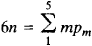# Statics of Mechanisms

The following article is from The Great Soviet Encyclopedia (1979). It might be outdated or ideologically biased.

## Statics of Mechanisms

the branch of the theory of machines and mechanisms that studies the methods of determining the reactions of the components of kinematic pairs under the condition that the inertial forces of elements of the mechanism may be disregarded. Unknown reactions are found from the equations of equilibrium of stationary bodies, that is, from the equations of statics. For the solution to be definite, it is necessary for the number of equilibrium equations to equal the number of unknowns to be determined. The number of equilibrium equations for an n-element three-dimensional kinematic chain, that is, a connected system of linkages forming kinematic pairs, is 6n. However, the number of unknowns to be determined in a calculation of forces depends on the number of coupling conditions imposed by each kinematic pair on the relative motion of elements of the pair. The number of coupling conditions of a kinematic pair is called the class number of the pair and varies from one to five. If the number of class 5 pairs, for example, rotating and transla-tional pairs, is designated by p5, the number of class 4 pairs, for example, cylindrical pairs, by p4, and so forth, then the condition for equality of the number of equilibrium equations and the number of unknowns assumes the formA group of linkages and kinematic pairs that satisfy this condition is called a statically determined group or an “Assur group,” after the Russian scientist who proposed the principle of dividing a mechanism into such groups and fundamental linkages. In the absence of frictional forces, the system of equilibrium equations of such a group is linear in the unknowns to be determined. An analogous system of equations is obtained by taking into account the inertial forces of the linkages according to d’Alembert’s principle. The methods of the statics of mechanisms therefore coincide with the methods of the dynamic force analysis of mechanisms.